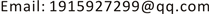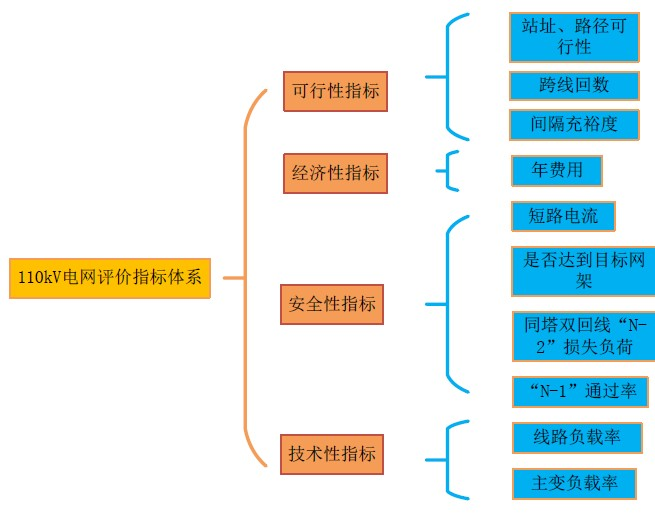﻿ 基于层次分析法的电网网架结构综合评价模型研究 Research on Comprehensive Evaluation Model of Grid Structure Based on Analytic Hierarchy Process

Journal of Electrical Engineering
Vol. 06  No. 03 ( 2018 ), Article ID: 26889 , 8 pages
10.12677/JEE.2018.63030

Research on Comprehensive Evaluation Model of Grid Structure Based on Analytic Hierarchy Process

Peipei Li, Zhenghua Chen, Jie Deng, Hao Chen

State Grid Yangzhou Power Supply Company, Yangzhou JiangsuReceived: Aug. 29th, 2018; accepted: Sep. 13th, 2018; published: Sep. 20th, 2018ABSTRACT

Grid structure planning is an important link of power grid construction. The reasonable grid is an effective means to guarantee the safety, stability and economic operation of power grid, and also an important prerequisite to guarantee the reasonable, effective and full utilization of power grid construction funds. This paper mainly takes Yangzhou 110 kV grid structure as an example and adopts the analytic hierarchy process (AHP) to establish the comprehensive evaluation model of grid structure. This method includes four indicators: technical, safety, economic and ease of implementation. This model aims to eliminate subjective factors in the evaluation process and provide a reasonable evaluation method of the grid structure. It plays an important role in improving the adaptability of the grid planning scheme, grasping the development direction of the grid and increasing the economic and social benefits of the grid.

Keywords:Grid Structure, Evaluation Model, Optimize, Hierarchy Process (AHP)1. 引言

110 kV电网网架结构的确定作为配电网规划的主体与很多因素密切相关，应根据技术性、安全性、经济性和实施难易程度等综合因素合理选择并具体确定。目前，江苏省电网网架规划中，对电网各相关因素以定性分析为主，经验性较强，同时难以分析各因素对网架结构的影响程度。主要原因是目前高压电网网架的确定缺少合理且具有较强实用性的综合评价体系  ，导致网架的确定无法实现定量计算，网络结构的选择随意性较大。

2. 扬州电网基本情况

3. 电网综合评价体系

3.1. 评价指标

3.1.1. 技术性指标

${\lambda }_{Ti}=\frac{{P}_{Ti}}{{S}_{Ti}}×100%$ (1)

${\lambda }_{Li}=\frac{{P}_{Li}}{{S}_{Li}}×100%$ (2)

3.1.2. 安全性指标

① “N − 1”通过率PN1Figure 1. The evaluation index system construction of the 110 kV grid frame

${P}_{N-1}=\frac{{n}_{N-1}}{N}×100%$ (3)

② 同塔双回线“N − 2”情况下的损失负荷ΔP1

③ 是否达到目标网架

110千伏电网应充分利用现有电网结构的资源，因地制宜，因网制宜，逐步形成以链式结构为主的目标网架，减少辐射方式供电。

④ 短路电流

3.1.3. 经济性指标

${F}_{_ann}={F}_{_tot}×\eta +{F}_{_op_ann}$ (4)

${F}_{_tot}={F}_{_T_tot}+{F}_{_L_tot}$ (5)

$\eta =\frac{i{\left(1+i\right)}^{y}}{{\left(1+i\right)}^{y}-1}$ (6)

${F}_{_op_ann}={F}_{_con_ann}+{F}_{_los}$ (7)

${F}_{_con_ann}=0.042×{F}_{_T_tot}+0.022×{F}_{_L_tot}$ (8)

${F}_{_los}=a×{W}_{_ann}$ (9)

${W}_{_ann}=\Delta P×t$ (10)

3.1.4. 可行性指标

3.2. 指标得分标准

3.3. 评估指标权重

3.4. 综合模型评分

${A}_{j}^{m+1}=\sum _{i-1}^{n}{A}_{i}^{m}{W}_{i}^{m}$ (11)

4. 层次分析法

1) 指标分层

2) 构造判别矩阵

$X=\left[\begin{array}{cccc}{x}_{11}& {x}_{12}& \cdots & {x}_{1n}\\ {x}_{21}& {x}_{22}& \cdots & {x}_{2n}\\ ⋮& ⋮& {x}_{ij}& ⋮\\ {x}_{n1}& {x}_{n2}& \cdots & {x}_{nn}\end{array}\right]$ (12)

3) 一致性检验

$\text{CI}=\frac{\lambda -n}{n-1}$ (13)Table 3. The standard value of the stochastic consistency index

$\text{CR}=\frac{\text{CI}}{\text{RI}}$ (14)

4) 计算权重向量

5. 算例分析Table 4. The weight of each index in the comprehensive evaluation system

6. 总结

Research on Comprehensive Evaluation Model of Grid Structure Based on Analytic Hierarchy Process[J]. 电气工程, 2018, 06(03): 256-263. https://doi.org/10.12677/JEE.2018.63030

1. 1. 张绕钢. 怒江电网110kV网架结构优化研究[J]. 中国电业(技术版), 2011(10): 25-27.

2. 2. 周瑞臣, 王宏, 李秀花. 廊坊110kV电网存在的主要问题及对策[J]. 华北电力技术, 2007(S1): 43-45.

3. 3. 谭伟球. 基于110KV电网网架结构优化研究[J]. 中国新技术新产品, 2011(7): 139-140.

4. 4. 史雨春, 曾亮. 110kV电网目标网架结构适应性分析[J]. 湖北电力, 2010, 34(4): 40-41.

5. 5. 秦梁栋, 宋静, 徐海东, 等. 河北南网高压电网网架综合评价方法探讨[J]. 电力系统及其自动化学报, 2011, 23(3): 89-94.